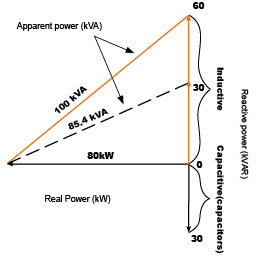COVID-19 Update: When delivering all energy efficiency programs, your health and safety is our priority. Learn more.

# Industrial Customers Cut Costs by Raising Power Factor

A low power factor causes voltage drops and energy losses on the system, making it necessary to oversize all the facilities from the generating station to your electrical panel. By improving power factor, you can reduce your demand charge and improve equipment efficiency.

## WHAT IS POWER FACTOR?

Power factor is the ratio between active power and apparent power. The power supplied by us is called apparent power (kVA). Apparent power is broken down into active power (kW), and reactive power (kVAR). Active power provides energy for motion, heat, light and sound. Reactive power (inductive) is used to create magnetic fields necessary to drive rotating equipment such as motors, compressors, etc.

Power factor = Active power (kW) X 100 / Apparent power (kVA)

By installing capacitors, the reactive power component (kVAR) by the Utility will decrease causing the apparent power component (kVA) to decrease thereby improving the power factor.

Example:

Customer “A” has a load with the following characteristics:

Apparent power = 100 kVA

Active power = 80 kW

Reactive power = 60 kVAR

Power factor> = 80%

The installation of a 30 kVAR of capacitors will decrease the reactive power supplied by the Utility to 30 kVAR and the apparent power supplied by the Utility to 85.4 kVA. The power factor measured at the Utility meter increases to 80 kW / 85 kVA> X 100 = 94%.

The relation between the real power (kW), the apparent power (kVA) and the reactive power (kVAR) may be represented by a triangle as follows:With respect to “inductive loads”, a load that uses magnetic fields. EG: motors, relays, solenoids. One rule of thumb is that if it moves, it’s probably an inductive load.
Power used and needed to support the transfer of real power, but doesn’t do any real work. I.e.: power used to create magnetic fields in rotating equipment like motors. The unit used for reactive power is volt amps reactive (VAR).
Used to express reactive power in a circuit. 1000 Volt Amps Reactive (VAR) = 1 kiloVolt Amps Reactive (kVAR).
Usually used to express power in all forms, but is reserved to express real power. 1000 Watts (W) = 1 kiloWatt (kW).
Used to express the total load in a circuit. 1000 VA = 1 kVA (kilovolt Amps).
An electronic device capable of storing an electric charge. They usually consist of two conductors separated by an insulating material.
Power which is a combination of ‘real power’ and ‘reactive power’. The unit for apparent power is volt amps (VA).
Power used to perform mechanical work and operate resistive loads like heaters, and incandescent lights. The unit used for active power is Watts (W).
The charges the electrical utility put on your bill for peak demand. The demand charges are usually derived from peak kW or kVA.
The ratio of the real power flowing to the load to the apparent power in the circuit.